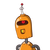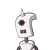# PQR is a triangle, right angled at Q. If PQ = 15m and PR = 17m then find QR.​

PQR is a triangle, right angled at Q. If PQ = 15m and PR = 17m then find QR.​

### 2 thoughts on “PQR is a triangle, right angled at Q. If PQ = 15m and PR = 17m then find QR.​”

1.QR = 8m is the right answer of this question

2.hyp² = side² + side²

17² = 15² + side²

289 = 225 + side²

side² = 289 – 225

side² = 64

side = √64

side = 8

.

.

.

QR= 8m

.

.

.

i hope it helps you

.

.

.

keep smiling always